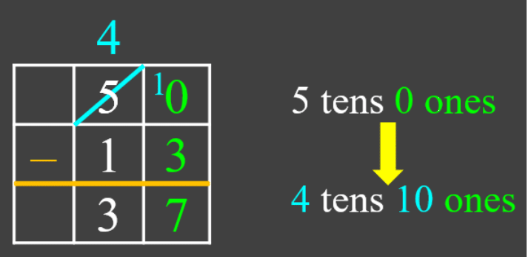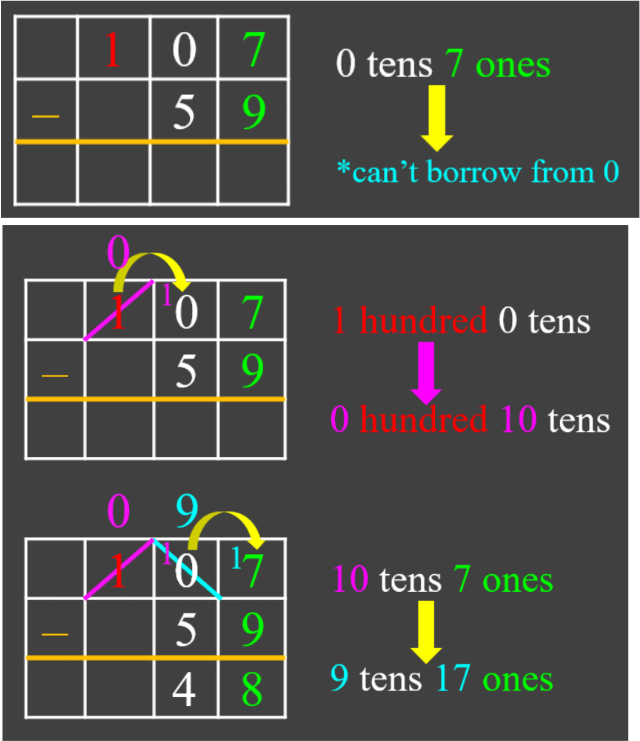# Subtracting multi-digit numbers#### Everything You Need in One Place

Homework problems? Exam preparation? Trying to grasp a concept or just brushing up the basics? Our extensive help & practice library have got you covered.#### Learn and Practise With Ease

Our proven video lessons ease you through problems quickly, and you get tonnes of friendly practise on questions that trip students up on tests and finals.#### Instant and Unlimited Help

Our personalized learning platform enables you to instantly find the exact walkthrough to your specific type of question. Activate unlimited help now!

0/2
##### Intros
###### Lessons
1. Introduction to Subtracting Multi-Digit Numbers:
2. Introduction to subtracting multi-digit numbers
3. Regrouping technique: borrowing when there are zeroes
0/17
##### Examples
###### Lessons
1. Subtracting 2-digit numbers with regrouping
Subtract:
1. 71 - 9
2. 50 - 13
3. 65 - 36
4. 94 - 48
2. Subtracting 3-digit numbers with regrouping
Subtract:
1. 468 - 281
2. 812 - 223
3. 950 - 671
3. Subtracting from 100 or 1000
Subtract:
1. 100 - 68
2. 100 - 49
3. 1000 - 712
4. 1000 - 543
4. Subtracting 4-, 5- and 6- digit numbers with regrouping
Subtract:
1. 5431 - 251
2. 76820 - 34951
3. 619687 - 418939
5. Subtracting three numbers
Subtract:
1. 695 - 203 - 318
2. 100 - 37 - 42
6. Subtracting multi-digit numbers with regrouping: word problem
Billy went to the electronics store with $2502. He bought a laptop for$1599. How much money does he have left?
0%
##### Practice
###### Topic Notes

In this lesson, we will learn:

• How to subtract examples with regrouping: from two 2-digit numbers up to two 6-digit numbers
• How to subtract three numbers
• How to regroup when there are zeroes

Notes:

• If you need to borrow when subtracting:
• 1 ten can be regrouped ("exchanged") for 10 ones
• 1 hundred can be regrouped for 10 tens
• 1 thousand can be regrouped for 10 hundreds
Example 1:Example 2: